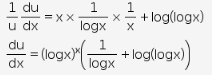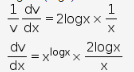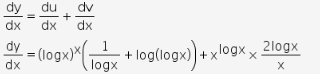# Find dy/dx y = (log x)x + (x)log x mention each and every formula and minute details

find dy/dx
y = (log x)x + (x)log x
mention each and every formula and minute details

y = (logx)x + xlogx
y = u + v
u = (logx)x
Taking log on both sides,
log u = xlog(logx)v = xlogx
Taking log on both sides,
logv = logx × logx
logv = (logx)2y = u + v